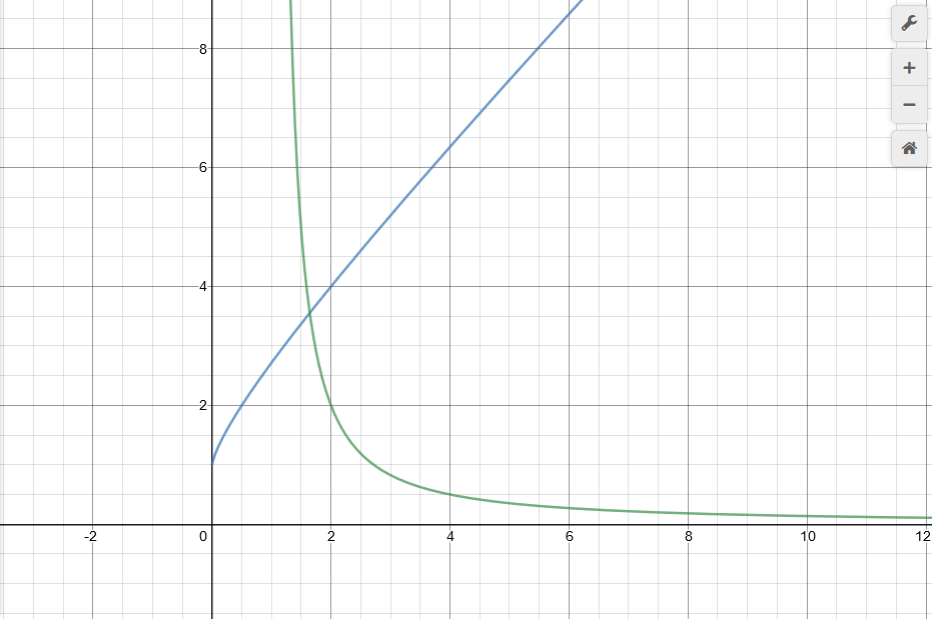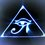# x^y = y^x

Trivial Solutions:

$1^{1}=1^{1}$

Based on some algebraic considerations solutions can be found:

$(x)^{(y)}= (y)^ {(x)}$

$assumption: y= kx$

$(x)^{(kx)}= (kx)^ {(x)}$

$(x)^{(\frac{kx}{x})}= (kx)^ {(\frac{x}{x})}$

$(x)^{(k)}= (kx)^ {(1)}$

$(x)^{(k)}= (kx)$

$(\frac{x^{(k)}}{x})= (\frac{kx}{x})$

$(x)^{(k-1)}= (k)$

$x= (k)^{(\frac{1}{k-1})}$

$x= \sqrt[k-1]{k}$

$y= k \sqrt[k-1]{k}$

$(\sqrt[k-1]{k})^{(k\sqrt[k-1]{k})}= (k\sqrt[k-1]{k})^ {(\sqrt[k-1]{k})}$

ex1.

When we let $k=2$ we get $y=4$ and $x =2$. $2^{4} = 4^{2}$ $16=16$

When we let $k =\frac{1}{2}$ we get the inverse relationship where $x=4$ and $y=2$.

ex2.

When we let k=4 we get $x =\sqrt{4}$ and $y=4\sqrt{4}$

$(\sqrt{4})^{(4\sqrt{4})}= (4\sqrt{4})^ {(\sqrt{4})}$ 18.805... = 18.805...

When we let $k=\frac{1}{4}$ we get the inverse scenario where $x=4\sqrt{4}$ and$y =\sqrt{4}$

There are some restriction on $k$. The functions for $x$ and $y$ are undefined when $k=0$ since you it results in $x=0^{\frac{1}{-1}}=0^{-1}=\frac{1}{0}$ and $y=0*0^{\frac{1}{-1}}=0*\frac{1}{0}$ and since we can't divide by zero this is undefined. Interestingly though $0^{0}$ is defined as $0^{0} =1$ and therefore $0^{0}=0^{0}$. Although this is outside our solution set since no value of $k$ seems to produce this result. Other cases that are undefined include the case where $k=1$ since it again results in a division by zero. The solution $1^{1}=1^{1}$ is also outside this solution set since there is no value of $k$ that produces one for $x$ and one for $y$. You will find that k can also not be a negative number since it results in negative roots and that is an operation that is undefined.

ex 3.

When we let $k=-1$ we get $y= -1 \sqrt[-1-1]{-1}= -1 \sqrt[-2]{-1}= undefined$ and $x= \sqrt[-1-1]{-1}= \sqrt[-2]{-1}= undefined$

For a similar reason k can not be a complex number. Complex numbers also produce undefined results using this method. Taking a complex root is undefined.

If we look at a graph of the equation for x and y we can see this clearer. $y$ is in blue and $x$ is in green. As we can see $x$and $y$ have the same domain for $k$ but they have different ranges. The one sided limit for $y$ as k approaches zero appears to be one. That means as k gets smaller and smaller y will get closer to one. On the other hand if we consider what happens to x it seems to behave in the opposite manner. It apporaches infinity as k approaches zero. This explains the behavior we saw earlier with the inverses of k. As k changes x and y have to swap places. If y starts out larger than x and we decrease y eventually y will equal x. We find at k=1.643 x=y =3.556. As y decreases x will increase to maintain the relationship. Unfortunately the fact that x and y do not have the same range means that y can not be less than one but x can which is not exactly true because we know we can just flip x and y and the equation will still look the same. So we know simply by looking at the graph of x and y that this does not include the full solution set.Note by Brody Acquilano
6 months ago

This discussion board is a place to discuss our Daily Challenges and the math and science related to those challenges. Explanations are more than just a solution — they should explain the steps and thinking strategies that you used to obtain the solution. Comments should further the discussion of math and science.

When posting on Brilliant:

• Use the emojis to react to an explanation, whether you're congratulating a job well done , or just really confused .
• Ask specific questions about the challenge or the steps in somebody's explanation. Well-posed questions can add a lot to the discussion, but posting "I don't understand!" doesn't help anyone.
• Try to contribute something new to the discussion, whether it is an extension, generalization or other idea related to the challenge.

MarkdownAppears as
*italics* or _italics_ italics
**bold** or __bold__ bold
- bulleted- list
• bulleted
• list
1. numbered2. list
1. numbered
2. list
Note: you must add a full line of space before and after lists for them to show up correctly
paragraph 1paragraph 2

paragraph 1

paragraph 2

[example link](https://brilliant.org)example link
> This is a quote
This is a quote
    # I indented these lines
# 4 spaces, and now they show
# up as a code block.

print "hello world"
# I indented these lines
# 4 spaces, and now they show
# up as a code block.

print "hello world"
MathAppears as
Remember to wrap math in $$ ... $$ or $ ... $ to ensure proper formatting.
2 \times 3 $2 \times 3$
2^{34} $2^{34}$
a_{i-1} $a_{i-1}$
\frac{2}{3} $\frac{2}{3}$
\sqrt{2} $\sqrt{2}$
\sum_{i=1}^3 $\sum_{i=1}^3$
\sin \theta $\sin \theta$
\boxed{123} $\boxed{123}$

Sort by:

Is there any restriction for $k$?

- 6 months ago

Just as another visualization aid:

If $x,y>0,$ you can take the log of both sides, and get $\frac{\ln x}{x} = \frac{\ln y}{y}.$ Drawing horizontal lines on the graph of $\frac{\ln x}{x}$ shows that for any positive value of $z \le 1/e,$ there are exactly two values $x,y$ with $\frac{\ln x}{x} = \frac{\ln y}{y} = z.$

- 6 months ago

Wow, I had been wondering this for a long time. Thanks!

- 6 months ago

I have updated the note and will continue to update it as my knowledge on this subject improves. I have included some of the restriction on k. Although I by no means speak authoritatively on this subject. I also have been wondering this for a long time and only recently stumbled upon this solution.

- 6 months ago

If anyone has a better solution or more complete solution please indulge me.

- 6 months ago

I concur. I noticed a similar result with the intersections of exponential growth functions and polynomial functions. i.e. x^2 = 2^x. One function is always exponential,either growth or decay, and the other should be a polynomial ,of even or odd degree (which affects end behavior). I didn't exactly try every combination but for the ones I tried I noticed two intersections.

- 5 months, 4 weeks ago

“0^0 is defined as 1” Respect!

- 5 months, 3 weeks ago

In fact, this is a transcendental equation, so you can't write the solution in the form

x = f(y)

for any simple function (closed-form) f. But let me tell you how you would be best advised to analyze this equation:

First take the log of both sides:

log(X^Y) = log(Y^X)

and simplify:

Ylog(X) = Xlog(Y)

and then divide by X*Y:

log(X) log(Y) ------ = ------. X Y

Now you should consider the function

     log(x)


f(x) = ------. x

Clearly, we have a solution to the last equation if and only if

f(X) = f(Y).

Well, this happens when X = Y, but does it happen elsewhere? If we graph

y = f(x)

we will find that f increases from y = -infinity at x = 0 to y = 1/e at x = e (that's e = 2.71828... whether you used the common log or the natural log or the log to any other base), and then f decreases from y = 1/e at x = e to y = 0 at x = infinity.

Well, if X and Y are different values and

f(X) = f(Y),

then that means that there is a horizontal line which passes through our function at two points (namely X and Y). Look at the function, and you'll find that the smaller value is somewhere between 1 and e, and the larger value is bigger than e. Also, the closer the smaller value is to e, the closer the larger value is to e. The closer the smaller value is to 1, the bigger the larger value is.

So what you find is that if X <= 1, then the only solution is Y = X. Similarly, if X = e, then the only solution is Y = X. But if

1 < X < e,

then there are exactly two solutions for Y, one of which is Y = X, and the other is some number bigger than e. Similarly, if

e < X,

then there are exactly two solutions for Y, one of which is Y = X, and the other is some number between 1 and e.

But can you write out a formula for the smaller value in terms of the bigger value, or vice-versa? Well, not using any closed-form function. But you can use numerical methods to find approximate solutions for any X value.

When I was doing some homework help, I got this problem and came up with this solution.

- 4 months ago